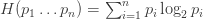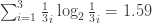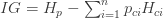Exploring a new dataset is all about generally getting to know your surroundings, understanding the data structure, understanding ranges and distributions, and getting a sense of patterns and relationships. Suppose you’re exploring a new dataset on customer churn. You may also be interested in exploring which variables provide the most information about whether or not a customer has churned. In this post I’ll talk a bit about how to use Shannon Entropy and Information Gain to help with this.

To keep things simple, we’ll explore the Iris dataset (measurements in centimeters for 3 species of iris).

##   Sepal.Length Sepal.Width Petal.Length Petal.Width Species
## 1          5.1         3.5          1.4         0.2  setosa
## 2          4.9         3.0          1.4         0.2  setosa
## 3          4.7         3.2          1.3         0.2  setosa
## 4          4.6         3.1          1.5         0.2  setosa
## 5          5.0         3.6          1.4         0.2  setosa
## 6          5.4         3.9          1.7         0.4  setosa

Which of these 4 features provides the “purest” segmentation with respect to Species? Or to put it differently, if you were to place a bet on the correct species, and could only ask for the value of 1 measurement, which one would give you the greatest likelihood of winning your bet?

For starters, let’s define what we mean by Entropy and Information Gain.

# Shannon Entropy

Entropy, as it pertains to information theory, tells us something about the amount of knowledge we have about a given set of things (in this case, our set of things would the Species of Iris). By “knowledge” here I mean how certain we are of what we would draw at random from the set. In fact, you can think of Entropy as having a perfect inverse relationship with knowledge, where the more knowledge we have, the lower the entropy. For an intuitive, detailed account (and an intuitive derivation of the formula below), check out Shannon Entropy, Information Gain, and Picking Balls from Buckets. In short, entropy provides a measure of purity. So how is Shannon Entropy defined?Where $$\ p_i$$ is the probability of value $$\ i$$ and $$\ n$$ is the number of possible values.

Suppose we took a subset of the iris data containing only the Setosa species:

setosa_subset <- iris[iris$Species=="setosa",] And were then interested in how pure the Species feature is, using the above definition of Shannon Entropy. Intuitively, we know we have 100% knowledge of the Iris Species, since we only have Setosa. Therefore, we must be at the lower bound of entropy. Let’s create a function to compute entropy, and try it out. #compute Shannon entropy entropy <- function(target) { freq <- table(target)/length(target) # vectorize vec <- as.data.frame(freq)[,2] #drop 0 to avoid NaN resulting from log2 vec<-vec[vec>0] #compute entropy -sum(vec * log2(vec)) } entropy(setosa_subset$Species)
##  0

As expected, we see that our entropy is indeed 0, indicating that we have complete knowledge of the contents of this set. If we were asked to draw one observation at random and predict the species, we know we’d draw a Setosa.

However, in the iris dataset we actually have 3 species of Iris (Setosa, Versicolor, Virginica), each representing 1/3 of the data. Therefore, we’d expect a higher entropy if we include the complete data. If you imagine a bucket filled with differently coloured balls representing the different species of Iris, we now have less knowledge about what colour of ball (what species) we would draw at random from the bucket. We can use our entropy function again to see this.

entropy(iris$Species) ##  1.584963 As expected, we now see non-zero entropy. Note that with 3 classes of Species each equally likely to be drawn, the Shannon Entropy is defined as follows.# Information Gain Continuing with our iris example, we could ask the following: “Can we improve (reduce) the entropy of the parent dataset by segmenting on Sepal Length?” Information gain helps answer this question by measuring how much “information” a feature gives us about the class. The idea is to look at how much we can reduce the entropy of our parent node (in this case Species) by segmenting on a given child. Note that segmentation can be done on either categorical or numerical features (just like in a decision tree), but to continue with our Iris example, we’ll be looking at IG on numerical features. Information Gain is defined as follows:Where $$\ H_p$$ is the entropy of the parent (the complete, unsegmented dataset), n is the number of values of our target variable (and hence the number of child segments), $$\ p_{ci}$$ is the probability that an observation is in child $$\ i$$ (the weighting), and $$\ H_{ci}$$ is the entropy of child (segment) $$\ i$$. In this case, Sepal Length is numeric. For categorical variables, we simply segment on each possible value. In the numeric case, we will bin the data according to the desired number of breaks (which is set to 4 by default). If we segment using 5 breaks, we get 5 children. Note e is the computed entropy for this subset, p is the proportion of records, and n is the number of records in that child. #returns IG for numerical variables. IG_numeric<-function(data, feature, target, bins=4) { #Strip out rows where feature is NA data<-data[!is.na(data[,feature]),] #compute entropy for the parent e0<-entropy(data[,target]) data$cat<-cut(data[,feature], breaks=bins, labels=c(1:bins))

#use dplyr to compute e and p for each value of the feature
dd_data <- data %>% group_by(cat) %>% summarise(e=entropy(get(target)),
n=length(get(target)),
min=min(get(feature)),
max=max(get(feature))
)

#calculate p for each value of feature
dd_data$p<-dd_data$n/nrow(data)
#compute IG
IG<-e0-sum(dd_data$p*dd_data$e)

return(IG)
}

Working through the function, we can see that we first split the feature (Sepal Length in this case) into 5 bins. We then produce a summary table including entropy using dplyr, and calculate the proportion of each records in each bin. Let’s look at the dd_data table below:

##   cat        e  n min max
## 1   1 2.653326 32 4.3 5.0
## 2   2 2.645348 41 5.1 5.7
## 3   3 2.735014 42 5.8 6.4
## 4   4 2.486441 24 6.5 7.1
## 5   5 2.299896 11 7.2 7.9

We now have everything we need to compute IG. We simply compute the entropy of the root node (Species) using 1.5849625, then subtract the sum of the bin entropies weighted by the proportion of data they represent exactly as per the IG formula shown above.

IG_numeric(iris, "Sepal.Length", "Species", bins=5)
##  0.6402424

# Exploring data using Information Gain

So what do we know? Well, it seems that segmenting on Sepal Length does improve entropy over the complete, unsegmented dataset. That means we’re improving the information we have about what species we might draw at random if we segment by Sepal Length. But where does 0.6402424 stand comparatively? I’m going to do this super old school because, well, I’m lazy.

col_name<-character()
ig<-numeric()
features<-names(iris)
for (i in 1:4){
col_name[i]<-names(iris)[i]
ig[i]<-IG_numeric(iris, names(iris)[i], "Species", bins=5)
}
ig_df<-cbind(col_name, round(ig,2))

ig_df
##      col_name
## [1,] "Sepal.Length" "0.64"
## [2,] "Sepal.Width"  "0.39"
## [3,] "Petal.Length" "1.27"
## [4,] "Petal.Width"  "1.32"

While we capture some information out of each variable, it looks like Petal Width actually provides the greatest information gain. In other words, If I had to make a bet on the Species of an Iris, with the help of only a single measurement, I’d chose Petal Width. Let’s again produce a summary table, but this time using the Petal Width variable and include the proportion species that fall into each bin.

data<-iris
data$cat<-cut(data[,"Petal.Width"], breaks=5, labels=c(1:5)) dd_data <- data %>% group_by(cat) %>% summarise(e=entropy(Petal.Width), n=length(Petal.Width), min=min(Petal.Width), max=max(Petal.Width), Setosa=length(Species[Species=="setosa"])/50, Versicolor=length(Species[Species=="versicolor"])/50, Virginica=length(Species[Species=="virginica"])/50) dd_data<- data.frame(dd_data) dd_data ## cat e n min max Setosa Versicolor Virginica ## 1 1 1.7005454 49 0.1 0.5 0.98 0.00 0.00 ## 2 2 0.5435644 8 0.6 1.0 0.02 0.14 0.00 ## 3 3 2.1504839 41 1.1 1.5 0.00 0.76 0.06 ## 4 4 2.0945684 29 1.6 2.0 0.00 0.10 0.48 ## 5 5 2.1855429 23 2.1 2.5 0.00 0.00 0.46 So it’s not perfect, but you can see that Petal Width is indeed quite telling. Again with the betting example if you tell me the Petal Width is between .1 and .5, I can say with certainty the species is Setosa. # Categorical variables While we’ve focused on numeric examples, Entropy and IG work just as well for categorical variables. In this case, we wouldn’t need to define the number of bins as we did in the numerical case. Have a look at the function below and feel free to try it out with your own data. #returns IG for categorical variables. IG_cat<-function(data,feature,target){ #Strip out rows where feature is NA data<-data[!is.na(data[,feature]),] #use dplyr to compute e and p for each value of the feature dd_data <- data %>% group_by_at(feature) %>% summarise(e=entropy(get(target)), n=length(get(target)) ) #compute entropy for the parent e0<-entropy(data[,target]) #calculate p for each value of feature dd_data$p<-dd_data$n/nrow(data) #compute IG IG<-e0-sum(dd_data$p*dd_data\$e)

return(IG)
}

I’ll include a quick example here using the airquality dataset. We’d likely want to bin the temps to do this more effectively, but in this case we’ll treat each temp seperately.

IG_cat(airquality, "Month", "Temp")
##  1.085021